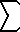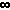Home    |    Teacher    |    Parents    |    Glossary    |    About UsEmail this page to a friendResources· Cool Tools · Formulas & Tables · References · Test Preparation · Study Tips · Wonders of MathSearchUnproved Theorems/b> (Math | Miscellaneous | Unproved)Riemann Hypothesis

zeta(s) = 1/1s + 1/2s + 1/3s + ... (s = a + it) all 0's of zeta(s) in strip 0<=a<=1 lie on central line a=1/2

Twin Primes occur infinitely

Twin primes are primes that are 2 integers apart. Examples include 5 & 7, 17 & 19, 101 & 103

Goldbach's Postulate

Every even # > 2 can be expressed as the sum of 2 primes.
4=2+2, 6=3+3, 8=3+5, 10=5+5, 12=5+7, .. , 100=3+97, ...

Euclid's Parallel Postulate

Through a point, not on a line, there exists exactly 1 line parallel to the given line. (Then there's those non-Euclidean people...)(k=1..) 1/kn = ?

Although others have found that this expression equals PI2 / 6 when n=2, PI4 / 90 when n = 4 and similar solutions for all possible even values of n, no one has discovered an exact value when n is an odd integer (3, 5, 7, ...) (note: when n=1, the sum does not converge, but it does has relations to the gamma constant).